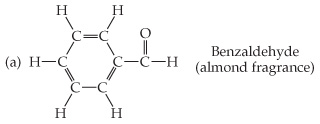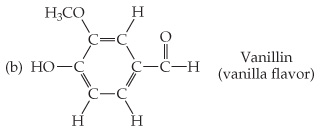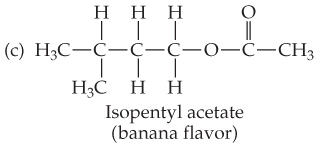# Problem: Based on the following structural formulas, calculate the percentage of carbon by mass present in each compound.Isopentyl acetate (banana flavor)

###### FREE Expert Solution

Mass % C:

• molar mass C7H14O2 = 130.18 g/mol###### Problem Details

Based on the following structural formulas, calculate the percentage of carbon by mass present in each compound.Isopentyl acetate (banana flavor)